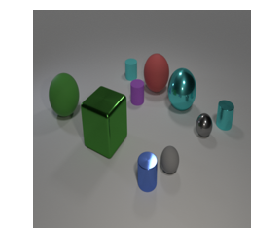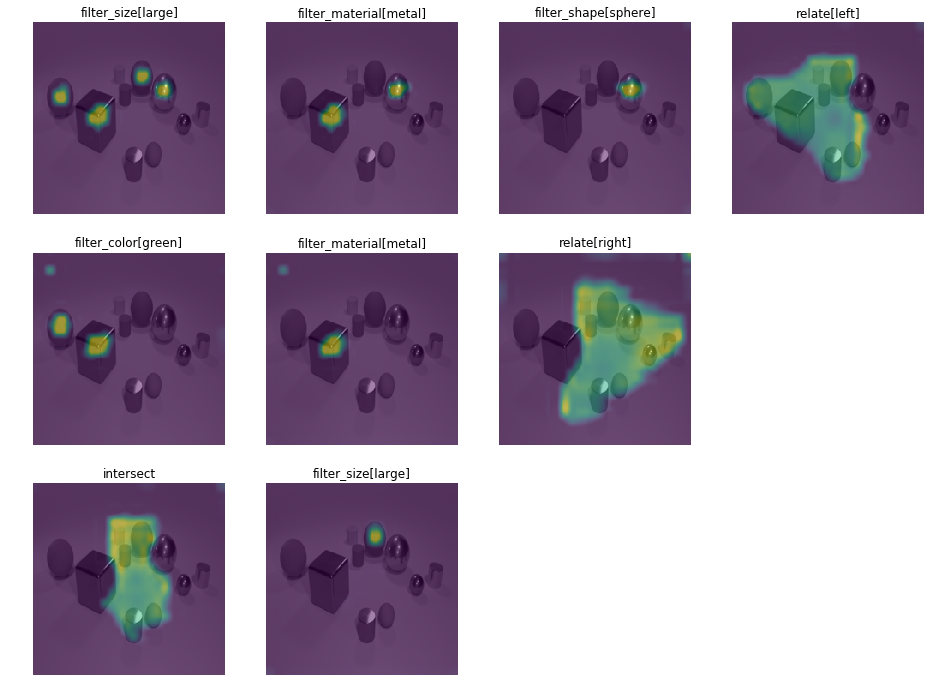This notebook illustrates the entire VQA pipeline using a TbD-Net. It loads the appropriate models necessary for performing the task, then allows a user to input a natural-language question and provide an image, get an answer back, and view intermediary attention masks.

In :
from pathlib import Path
from itertools import groupby

import numpy as np
from scipy.misc import imresize
import matplotlib.pyplot as plt
%matplotlib inline



Now let's make sure we have all the models we need, and download them if not. We'll also load all of them here.

Note: if you do not have our program generator model, our TbD-Net model, and PyTorch's ResNet-101 model, these will all be downloaded.

In :
vocab = load_vocab(Path('data/vocab.json'))

model_path = Path('./models')
program_generator_checkpoint = 'program_generator.pt'

tbd_net_checkpoint = 'clevr-reg-hres.pt'
if not (model_path / tbd_net_checkpoint).exists():

tbd_net = load_tbd_net(model_path / tbd_net_checkpoint, vocab)

In :
def run_question_and_image(question, img_path):
''' Utility function to forward a question and image through a TbD-Net model
and return the image and the intermediate attention masks.

Parameters
----------
tbd_net : torch.nn.Module
The TbD-Net module to forward the question and image through.

question : str
A natural-language question to produce the answer to.

img_path : Union[pathlib.Path, str]
The path to the image to use to answer question.

Returns
-------
Tuple[numpy.ndarray, str, List[Tuple[str, numpy.ndarray]]]
A tuple of (img, answer, [(operation, attention), ...])
Note that some of the intermediates will be None rather than a tuple, which
indicates a break in the logic chain. For example, in the question:
"What color is the cube to the left of the sphere and right of the cylinder?"
We have 3 distinct chains of reasoning. We first localize the sphere and look left.
We then localize the cylinder and look right. Thirdly, we look at the intersection
of these two, and find the cube. We'll encounter a None at the beginning of the
question and after the left and right operations.
'''
prog_var = generate_single_program(question, program_generator, vocab)
img, feat_var = extract_image_feats(img_path, feature_extractor)


There's one more function to set up. We want to be able to visualize our intermediate attention masks, so we define a function that takes the intermediaries from our run_question_and_image function above and displays the attention masks in a logical structure.

In :
def display_tree(intermediaries, interp='lanczos', img=None):
''' Convenience function for displaying intermediary outputs in as tree-like a
structure as it's worth computing.

Parameters
----------
intermdiaries : List[Tuple[str, numpy.ndarray]]
The [(operation, attention), ...] list to display.

interp : str
The type of interpolation to use. In our paper, we use lanczos interpolation
for the smoothed images and nearest for the rest of our visualizations.
Legal options are: (‘nearest’, ‘lanczos’, ‘bilinear’, ‘bicubic’, ‘cubic’).

img : numpy.ndarray, shape=(r, c, 3), optional (default=None)
The image to display under each attention mask.

Returns
-------
Tuple[matplotlib.figure.Figure, matplotlib.axes.Axes]
The figure and axes objects the visualization is displayed on.
'''
if img is not None:
color_to_gray = [0.2126, 0.7152, 0.0722] # luminance-preserving transform
gray = np.dot(imresize(img, (224, 224), 'bicubic'), color_to_gray)

num_rows = intermediaries.count(None) # None indicates a break in logic
x = intermediaries
# get the maximum run between None entries, which is the max number of columns
num_cols = max(len(tuple(j)) for _, j in groupby(x, key=lambda x: x is None))

# create our plots
fig, ax = plt.subplots(num_rows, num_cols, figsize=(4*num_cols, 4*num_rows),
squeeze=False)

row, col = 0, 0
intermediaries = intermediaries[1:] # the first operation (index 0) is always None
for i, intermediate in enumerate(intermediaries):
if intermediate is None:
while col < num_cols:
# remove all the axes that don't have a plot so we don't
# display annoying white boxes
ax[row, col].remove()
col += 1
row += 1
col = 0
continue
ax[row, col].set_title(intermediate)
interp = interp if interp else 'nearest'
if img is not None:
# if the user provides an image to underlay, display it with
ax[row, col].imshow(gray, cmap='gray')
ax[row, col].imshow(imresize(intermediate, (224, 224), interp), alpha=0.5)
else:
# otherwise just display the attention mask
ax[row, col].imshow(imresize(intermediate, (224, 224), interp))
ax[row, col].axis('off')
col += 1
while col < num_cols:
ax[row, col].remove()
col += 1
return (fig, ax)


In the cell below, provide your own question and image!

In :
img_path = Path('data/test_im_000012.png')
question = ' '.join(['What color is the big object that is left of the large metal sphere',
'and right of the green metal thing?'])
img, prediction, intermediaries = run_question_and_image(question, img_path)


Let's visualize the outputs to see where our model was looking during each stage of the reasoning process.

In :
fig, ax = plt.subplots()
ax.imshow(img)
ax.axis('off')

fig, ax = display_tree(intermediaries, interp='lanczos', img=img)Was the model right?

In :
prediction

Out:
'red'

Yes!